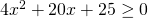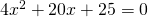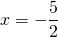# Solution assignment 06 Quadratic inequalities

### Assignment 6

Solve:### Solution

First we solve the corresponding equation:The left-hand side of this equation is a special product and can be written as:The solution of this equation is:The graph of the function in the left-hand side of the inequality is an 'opens up' parabola and has only one point in common with the-axis.
The solution of the equationsatisfies the inequality.